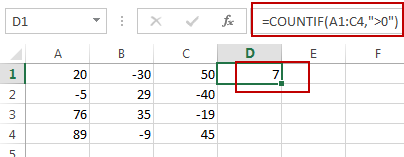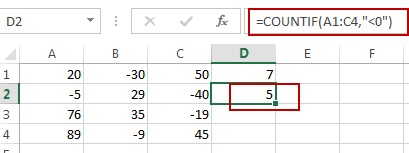# How to Count Positive or Negative Numbers in Excel

Whether you are dealing with financial data, scientific data, or any other type of numerical data, it’s crucial to be able to determine how many of the values in your dataset are positive or negative. Excel provides various built-in functions and tools to make this task quick and easy, allowing you to count the number of positive or negative values in a range of cells.

This post will guide you how to count cells that contain positive or negative numbers in Excel. How do I count positive and negative values with a formula in Excel. How to count all negative number or positive number in a given range of cells in Excel.

## 1. Count Only Positive Numbers in Excel

If you want to count the number of cells that contain only positive numbers in a range of cells in Excel, you can create a formula based on the COUNTIF function.
For example, you have a range of cells (A1:C4) that contain negtive and positive numbers, and you want to count only positive numbers, you can use the following formula:

`=COUNTIF(A1:C4,">0")`

Type this formula into a cell, and press Enter key.

###You will see that the number of positive values in the given range will be counted.

The COUNTIF function will count the number of cells in the given range that match the supplied criteria. You just need to replace `A1:C4 `with the range of cells you want to count. And “`>0`” means that it will count only values greater than zero, which are positive numbers.

## 2. Count Only Negative Numbers in Excel

If you want to count the number of only negative numbers in the range of cells A1:C4, just use the following formula:

`=COUNTIF(A1:C4,"<0")`

###You can also use another formula based on the SUMPRODUCT function to count only negative numbers in the selected range in Excel. Just do the following steps:

Step 1: Select the cell where you want to display the result of the count.

Step 2: type the following formula:

`=SUMPRODUCT(--(A1:C4<0))`
Note: The double negative “” converts the boolean output of the comparison to a 1 or 0, and SUMPRODUCT sums these 1’s and 0’s to count the number of negative numbers.

The cell will now display the number of negative numbers in the selected range.

## 3. Count Number of Cells in Excel

If you want to count the number of cells in Excel, you can use the COUNTA function. Type the following formula based on the COUNTA function:

`=COUNTA(Range)`

You need to replace “`Range`” with the range of cells you want to count. For example, if you want to count the range `A1:C4`, just type the following formula in a blank cell:

`=COUNTA(C1:C4)`

## 4. Count Only Numbers in Excel

If you want to count only numbers in Excel, you can use the COUNT function, just type the following formula based on the COUNT function:

`=COUNT(A1:C4)`

Or you can use another formula to achieve the same result of counting only numbers in the selected range cells.

`=SUMPRODUCT(--(ISTEXT(A1:C4)=FALSE))`

## 5. If Cell Contains Negative Number

If you want to check if a cell contains a negative number in Excel, you can use the following formula:

`=IF(A1<0, "Negative", "Not Negative")`

This formula checks the value in cell A1 to see if it’s less than zero (i.e. negative). If it is, the formula will display “`Negative`“. If it’s not negative, the formula will display “`Not Negative`“.

You can replace “`A1`” with the cell reference of the cell you want to check. You can also modify the text that the formula displays when the cell contains a negative number or when it doesn’t.

For example, if you want the formula to display “`Yes`” when the cell contains a negative number and “`No`” when it doesn’t, you can use this formula:

`=IF(A1<0, "Yes", "No")`

## 6. Related Functions

• Excel COUNTIF function
The Excel COUNTIF function will count the number of cells in a range that meet a given criteria. This function can be used to count the different kinds of cells with number, date, text values, blank, non-blanks, or containing specific characters.etc.= COUNTIF (range, criteria)…